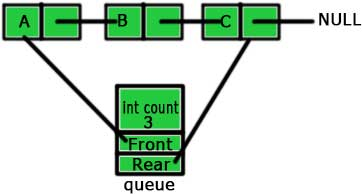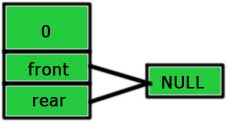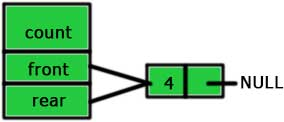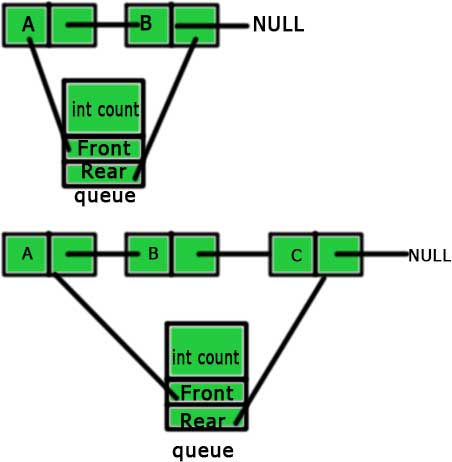# Making a queue using linked list in C

May 26, 2017 146976

### Previous:

The previous article was all about introducing you to the concepts of a queue. In this article, we will code up a queue and all its functions using a linked list.

The first thing required to make a queue using a linked list is obviously a linked list. So, let's start by creating a linked list.

struct node
{
int data;
struct node *next;
};
typedef struct node node;


The concepts and the codes of a linked list are explained in the article “Linked list in C”.

typedef struct node node – In this line of code, we are just representing struct node with node by using typedef. You can learn about typedef from the typedef chapter of the C course.

The next thing is to create a structure ‘queue’ which will store the front node, rear node and the total number of nodes in the linked list. This is similar to the picture given below:You can see that the structure ‘queue’ has three part – count, front and rear as discussed above. The value of the count, in this case, is 3 as there are total 3 nodes. You can also see that ‘front’ and ’rear’ are linked to the front and rear nodes respectively.

So, let’s make the structure of this queue.

struct queue
{
int count;
node *front;
node *rear;
};
typedef struct queue queue;


The next part is to initialize our queue and this will be done by making the count of the ‘queue’ 0 and pointing ‘rear’ and ‘front’ to NULL.So, let’s do this.

void initialize(queue *q)
{
q->count = 0;
q->front = NULL;
q->rear = NULL;
}


Till now, we are up to this:

struct node
{
int data;
struct node *next;
};
typedef struct node node;

struct queue
{
int count;
node *front;
node *rear;
};
typedef struct queue queue;

void initialize(queue *q)
{
q->count = 0;
q->front = NULL;
q->rear = NULL;
}


We can also check whether our queue is empty or not. The ‘rear’ (or ‘front’) will be NULL for an empty queue. So, we can easily check whether a queue is empty or not by checking whether the ‘rear’ is NULL or not.

int isempty(queue *q)
{
return (q->rear == NULL);
}


The next and the most important operations on a queue are enqueue and dequeue. So, let’s create them.

### enqueue

The steps for the enqueue operations are:

1. Make a new node (node *tmp; tmp = malloc(sizeof(node))).
2. Give the ‘data’ of the new node its value (tmp -> data = value).
3. If the queue is empty then point both ‘front’ and ‘rear’ of the queue to this node (q->front = q->rear = tmp;).4. If it is not, then point the rear of the queue to this new node and then make this new node rear (q->rear->next = tmp; q->rear = tmp;).5. Increase the ‘count’ of ‘queue’ by 1.
void enqueue(queue *q, int value)
{
node *tmp;
tmp = malloc(sizeof(node));
tmp->data = value;
tmp->next = NULL;
if(!isempty(q))
{
q->rear->next = tmp;
q->rear = tmp;
}
else
{
q->front = q->rear = tmp;
}
q->count++;
}


The first step is to make a new node and we are doing the same by
node *tmp
tmp = malloc(sizeof(node))

The second step is to give ‘data’ of this new node its value and this we are doing with tmp -> data = value.

The third and the fourth steps are done by:
if(!isempty(q)) {  q->rear->next = tmp;  q->rear = tmp; } else {  q->front = q->rear = tmp; }
The fourth step is to increase the ‘count’ of the ‘queue’ by 1 and we are doing this by q->count++.

You must have understood the enqueue operation. So, let’s deal with the dequeue operation now.

### dequeue

In dequeue operation, we delete the front node and returns its value. In order to do so, we need to make the ‘front’ pointer point to the node next to the current front node but this will led the current front node inaccessible. So, we will first make a temporary pointer to the current front node and delete it using the ‘free’ function later. The steps for the dequeue operations are:

1. Make a temporary node.
2. Point this temporary node to the front node of the queue.
3. Store the value of ‘data’ of this temporary node in a variable.
4. Point the ‘front’ pointer to the node next to the current front node.
5. Delete the temporary node using the ‘free’ function.
6. Return the value stored in step 3.
int dequeue(queue *q)
{
node *tmp;
int n = q->front->data;
tmp = q->front;
q->front = q->front->next;
q->count--;
free(tmp);
return(n);
}


The code is very simple and just follows the steps mentioned above.

node *tmp – Step 1

tmp = q->front – Step 2

n = q->front->data – Step 3

q->front = q->front->next – Step 4

free(tmp) – Step 5

return n – Step 6

So, the overall code for a stack using linkedlist is:

#include <stdio.h>
#include <stdlib.h>
#define TRUE 1
#define FALSE 0
#define FULL 10

struct node
{
int data;
struct node *next;
};
typedef struct node node;

struct queue
{
int count;
node *front;
node *rear;
};
typedef struct queue queue;

void initialize(queue *q)
{
q->count = 0;
q->front = NULL;
q->rear = NULL;
}

int isempty(queue *q)
{
return (q->rear == NULL);
}

void enqueue(queue *q, int value)
{
if (q->count < FULL)
{
node *tmp;
tmp = malloc(sizeof(node));
tmp->data = value;
tmp->next = NULL;
if(!isempty(q))
{
q->rear->next = tmp;
q->rear = tmp;
}
else
{
q->front = q->rear = tmp;
}
q->count++;
}
else
{
printf("List is full\n");
}
}

int dequeue(queue *q)
{
node *tmp;
int n = q->front->data;
tmp = q->front;
q->front = q->front->next;
q->count--;
free(tmp);
return(n);
}

{
{
printf("NULL\n");
}
else
{
}
}

int main()
{
queue *q;
q = malloc(sizeof(queue));
initialize(q);
enqueue(q,10);
enqueue(q,20);
enqueue(q,30);
printf("Queue before dequeue\n");
display(q->front);
dequeue(q);
printf("Queue after dequeue\n");
display(q->front);
return 0;
}


### Next:

Liked the post?
Developer and founder of CodesDope.